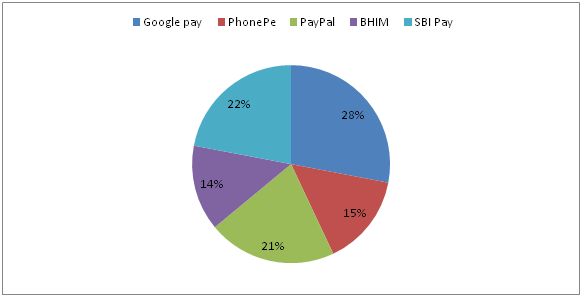# LIC AAO/SBI PO Prelims Quantitative Aptitude Questions 2019 (Day-10)

Dear Aspirants, Our IBPS Guide team is providing new series of Quantitative Aptitude Questions for LIC AAO/SBI PO 2019 so the aspirants can practice it on a daily basis. These questions are framed by our skilled experts after understanding your needs thoroughly. Aspirants can practice these new series questions daily to familiarize with the exact exam pattern and make your preparation effective.

[WpProQuiz 5630]

### Click Here for SBI PO Pre 2019 High-Quality Mocks Exactly on SBI Standard

Directions (Q. 1 – 5): what value should come in place of (?) in the following questions?

1) 25 % of ? – 1776 ÷ 12 – 2023 ÷ 7 = 52

a) 2178

b) 1842

c) 1735

d) 1956

e) None of these

2) 28 % of 1250 + (513/729) – (1/9) of 216 =? + 45

a) 412

b) 338

c) 284

d) 526

e) None of these

3) 4? × √225 = 48096 ÷ 8 + 9348

a) 5

b) 8

c) 10

d) 7

e) None of these

4) (37 × 9 + 17 × 6 – 23) ÷ [(8)2 + √324 + 21] = ?

a) 4

b) 8

c) 7

d) 5

e) None of these

5) 10 (¼) + 9 (¾) – 4 (3/8) – ? = 12 (7/8)

a) 2 (3/4)

b) 3 (5/6)

c) 4 (7/8)

d) 3 (¼)

e) None of these

Directions (Q. 6 – 10): Study the following information carefully and answer the given questions:

The following pie chart shows percentage distribution of total number people using 5 different payment apps in a certain state.6) If the total number of people using PayPal is 84000, then find the difference between the total number of people using Google Pay to that of PhonePe?

a) 48000

b) 56000

c) 52000

d) 44000

e) None of these

7) If the total number of people using digital payment app in the state is 400000, then find the average number of people using BHIM and SBI Pay?

a) 72000

b) 65000

c) 61000

d) 76000

e) None of these

8) Total number of people using PhonePe and BHIM together is approximately what percentage more/less than the total number of people using Google Pay and PayPal together?

a) 41 % more

b) 35 % less

c) 41 % less

d) 25 % more

e) 25 % less

9) Find the difference between the total number of people using PhonePe to that of BHIM app?

a) 11200

b) 7800

c) 9500

d) Can’t be determined

e) None of these

10) Find the ratio between the average number of people using Google pay and SBI pay together to that of the average number of people using PayPal and BHIM app together?

a) 13: 5

b) 10: 7

c) 25: 8

d) 21: 11

e) None of these

Direction (1-5) :

(25/100)*x – (1776/12) – (2023/7) = 52

(1/4)*x – 148 – 289 = 52

(1/4)*x = 52 + 148 + 289

(1/4)*x = 489

X = 489*4 = 1956

(28/100)*1250 + (513/9) – (1/9)*216 = x + 45

350 + 57 – 24 – 45 = x

X = 338

4x × 15 = (48096/8) + 9348

4x × 15 = 6012 + 9348

4x = 15360/15

4x = 1024

4x = 45

X = 5

(333 + 102 – 23) ÷ (64 + 18 + 21) = x

X = 412/103 = 4

10 (¼) + 9 (¾) – 4 (3/8) – 12 (7/8) = x

X = (10 + 9 – 4 – 12) (1/4 + ¾ – 3/8 – 7/8)

X = 3 (-2/8) = 3(-1/4)

X = (12 – 1)/4 = 11/4 = 2 (3/4)

Direction (6-10) :

The total number of people using PayPal = 84000

(21/100)*Total number of people using digital payments = 84000

Total number of people using digital payments

= > 84000*(100/21) = 400000

The difference between the total number of people using Google Pay to that of PhonePe

= > 400000*(28 – 15)/100

= > 52000

The total number of people using digital payment app in the state = 400000

The average number of people using BHIM and SBI Pay

= > 400000*[(14 + 22)/100]*(1/2)

= > 72000

Total number of people using PhonePe and BHIM together = 29 %

Total number of people using Google Pay and PayPal together = 49 %

Required % = [(49 – 29)/49]*100 = 40.81 % = 41 % less

Here, the total number of people using digital payments is not given. So, we can’t find the difference between the total number of people using PhonePe to that of BHIM app.

The average number of people using Google pay and SBI pay together

= > [(28 + 22)/100]*(1/2)

The average number of people using PayPal and BHIM app together

= > [(21 + 14)/100]*(1/2)

Required ratio = [(50/100)*(1/2)]: [35/100)*(1/2)]

= > 10: 7